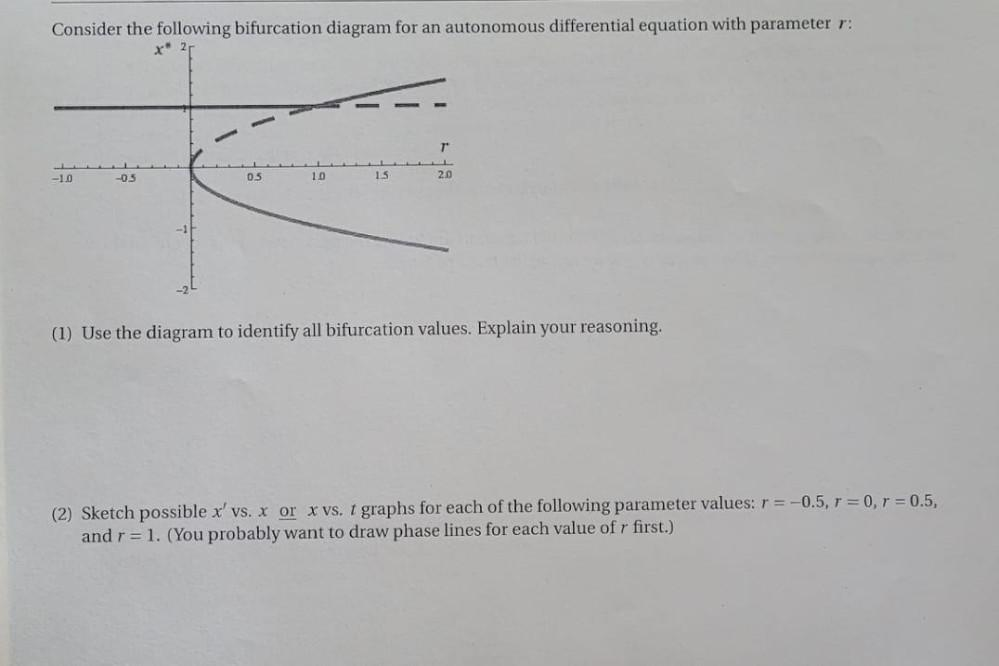Question:

# Consider the following bifurcation diagram for an autonomous differential equation with parameter r: T -1.0 -05 05 10 15 2.0 -1Consider the following bifurcation diagram for an autonomous differential equation with parameter r: T -1.0 -05 05 10 15 2.0 -1 (1) Use the diagram to identify all bifurcation values. Explain your reasoning. (2) Sketch possible x' vs. x or x vs. t graphs for each of the following parameter values: r = -0.5, r = 0, r = 0.5, and r = 1. (You probably want to draw phase lines for each value of r first.)